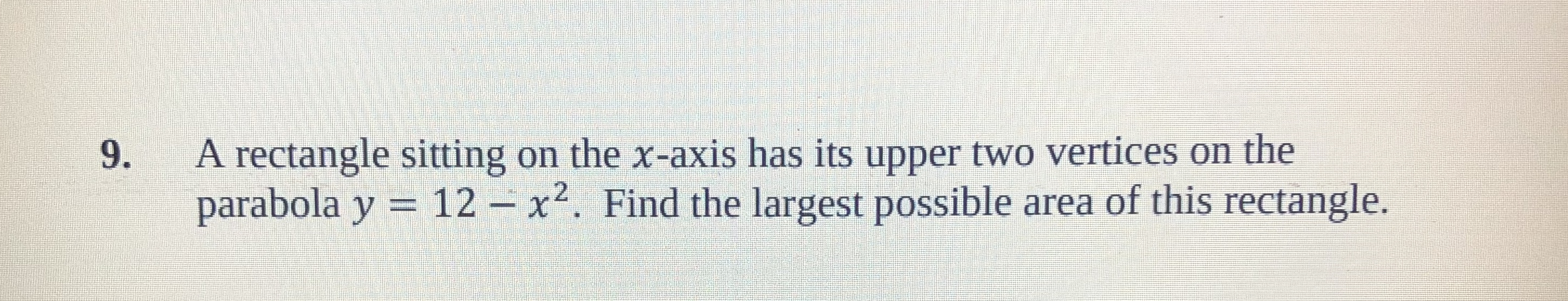# A rectangle sitting on the x-axis has its upper two vertices on theparabola y9.12x2. Find the largest possible area of this rectangle

Questionhelp_outlineImage TranscriptioncloseA rectangle sitting on the x-axis has its upper two vertices on the parabola y 9. 12x2. Find the largest possible area of this rectangle fullscreen
check_circle

Step 1

To calculate the largest possible area of the rectangle. If the rectangle is sitting on the x-axis and it two upper vertices is lies on the parabola y=12-x2. Now, first calculate the area function and then differentiate the function with respect to x and find the value of x and then substitute in value of y. After that substitute the value of x and y in the area function to calculate the area.

Step 2

The diagram for the provided information in the question is shown below,

Step 3

Now, the width of the rectangle is half the distance between point P due to axis of symmetry. Consider the width...

### Want to see the full answer?

See Solution

#### Want to see this answer and more?

Solutions are written by subject experts who are available 24/7. Questions are typically answered within 1 hour.*

See Solution
*Response times may vary by subject and question.
Tagged in

### Derivative